# JavaScript: Reducing the use of the For Loop

• aggregating a list objects into a single object
• transforming a list of objects into a list of different objects
• decreasing the size of a list
• side effects, such as the mutation of the original list
• potential multiple responsibilities in that bit of code

# Reduce: The ‘new’ basis of list processing

`const add2 = (a,b) => a + b// using the array methodconst sumList = list => list.reduce(add2, 0)// using a list processing libraryconst sumList = list => reduce(add2, 0, list) // in usageconst total = sumList(list)`
`let total = 0 ;for(let i = 0; i < list.length; i++) {    total = total + list[i] ;}`
`// ::  [a] -> aconst head = xs => xs// :: [a] -> [a]const tail = xs => xs.slice(1)// :: ( ((b,a) -> b), b, [a] ) -> bconst reduce = (f, agg, list) =>    (list.length == 0) ?        agg :        reduce(f, f(agg, head(list)), tail(list)) ;`
`// :: ( ((b,a) -> b), b, [a] ) -> bconst reduce = (f, agg, xs) => {    for(let i =0; i < xs.length; i++) {        agg = f(agg, xs[i])    }    return agg}`

# Map, Filter, Find is really just Reduce “Snazzed Up”

`// :: ([a], a) -> [a]const append = (xs, x) => xs.concat([x])// :: (a -> b) -> ([b], a) -> [b]const transformingAppender = f =>    (agg, x) =>        append(agg, f(x))// :: (a -> b) -> [a] -> [b]const map = f => reduce(transformingAppender(f), [])`
`// :: (a -> Boolean) -> ([a], a) -> [a]const conditionalAppender = f =>    (agg, x) =>        f(x) ?            append(agg, x) :            agg// :: (a -> Boolean) -> [a] -> [a]const filter = f => reduce(conditionalAppender(f), [])`

# Favor Readability over for-loop comfort

1. Abelson & Sussman, Structure and Interpretation of Computer Programs, ISBN-13: 978–0262510875
2. https://github.com/fantasyland/fantasy-land

--

--

--

## More from Todd Brown

A 25 year software industry veteran with a passion for functional programming, architecture, mentoring / team development, xp/agile and doing the right thing.

Love podcasts or audiobooks? Learn on the go with our new app.

## Managing Multiple Node.js Versions## Better Array check with Array.isArray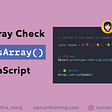## Generating some next generation web components## Learning Angular as a React Developer — Apiumhub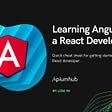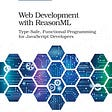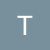## Todd Brown

A 25 year software industry veteran with a passion for functional programming, architecture, mentoring / team development, xp/agile and doing the right thing.

## Roman to Integer in Typescript (again :))## What is TypeScript?## Packaging a Typescript library — Part 1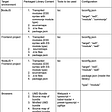## Get the First, Last and Middle Elements of an Array using JavaScript